Test: Geometry- 2

# Test: Geometry- 2

Test Description

## 15 Questions MCQ Test CSAT Preparation for UPSC CSE | Test: Geometry- 2

Test: Geometry- 2 for Quant 2022 is part of CSAT Preparation for UPSC CSE preparation. The Test: Geometry- 2 questions and answers have been prepared according to the Quant exam syllabus.The Test: Geometry- 2 MCQs are made for Quant 2022 Exam. Find important definitions, questions, notes, meanings, examples, exercises, MCQs and online tests for Test: Geometry- 2 below.
Solutions of Test: Geometry- 2 questions in English are available as part of our CSAT Preparation for UPSC CSE for Quant & Test: Geometry- 2 solutions in Hindi for CSAT Preparation for UPSC CSE course. Download more important topics, notes, lectures and mock test series for Quant Exam by signing up for free. Attempt Test: Geometry- 2 | 15 questions in 15 minutes | Mock test for Quant preparation | Free important questions MCQ to study CSAT Preparation for UPSC CSE for Quant Exam | Download free PDF with solutions
 1 Crore+ students have signed up on EduRev. Have you?
Test: Geometry- 2 - Question 1

### A cyclic quadrilateral is such that two of its adjacent angles are divisible by 6 and 10 respectively. One of the remaining angles will necessarily be divisible by:

Detailed Solution for Test: Geometry- 2 - Question 1

The two adjacent angles can be 30° and 60°, therefore others angle can be 150° and 120°

Test: Geometry- 2 - Question 2

### The volume of spheres are proportional to the cubes of their radii. Two spheres of the same material weigh 3.6 kg and 2.7 kg and the radius of the smaller one is 2 cm. If the two were melted down and formed into a single sphere, what would be its radius?

Detailed Solution for Test: Geometry- 2 - Question 2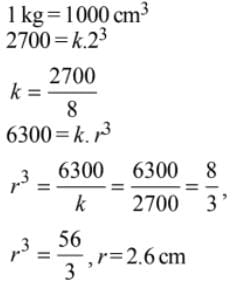Test: Geometry- 2 - Question 3

### Read the passage below and solve the questions based on it fix) is the area of a square where, Jr is the side of a square. g(x) is the perimeter of square where, Jr is the side of a square. h(xt y) is the area of a rectangle where* is the length and y is the breadth. i(xt y) is the perimeter of a rectangle where Jt is the length and y is the breadth.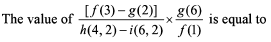Detailed Solution for Test: Geometry- 2 - Question 3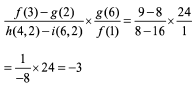Test: Geometry- 2 - Question 4

Read the passage below and solve the questions based on it.
A 10 m long piece of wire is cut into two pieces, one of which is bent into a circle and the other into the square enclosing it.

Q.

The ratio of the radius of the circle to the perimeter of the square is

Detailed Solution for Test: Geometry- 2 - Question 4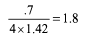Test: Geometry- 2 - Question 5

A square is inscribed in a semi circle of radius 10 cm. What is the area of the inscribed square? (Given that the side of the square is along the diameter of the semicircle.)

Detailed Solution for Test: Geometry- 2 - Question 5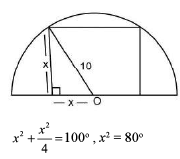Test: Geometry- 2 - Question 6

Two circles of an equal radii are drawn, without any overlap, in a semicircle of radius 2 cm. If these are the largest possible circles that the semicircle can accommodate, what is the radius (in cm) of each of the circles?

Detailed Solution for Test: Geometry- 2 - Question 6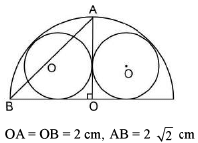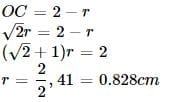Test: Geometry- 2 - Question 7

PQRS is a Trapezzium, in which PQ is Parralel to RS, and PQ = 3 (RS) . The diagnol of the Trapezzium intersect each other at X, then the ratio of,  ar ( ∆ PXQ)  : ar ( ∆ RXS)  is?

Detailed Solution for Test: Geometry- 2 - Question 7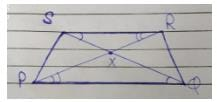In ∆ PXQ and ∆ RXS

=> angle P = angle R

angle Q  =  angle S

:- ∆ PXQ  ~ ∆ RXS  ( AA similarity rule)

ar ( ∆ PXQ) /  ar ( ∆ RXS) = ( PQ / RS) ^ 2

=  ( 3 / 1 ) ^ 2

=     9 / 1

Therefore,  ar ( ∆ PXQ) :  ar          ( ∆ RXS)

=   9:1

Test: Geometry- 2 - Question 8

Let ABCDEF be a regular hexagon. What is the ratio of the area of the triangle ACE to that of the hexagon ABCDEF?

Detailed Solution for Test: Geometry- 2 - Question 8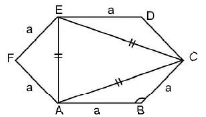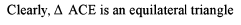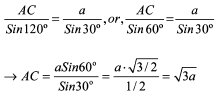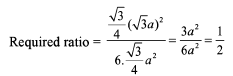Test: Geometry- 2 - Question 9

A pond 100 m in diameter is surrounded by a circular grass walk-way 2 m wide. How many square metres of grass is the on the walk-way?

Detailed Solution for Test: Geometry- 2 - Question 9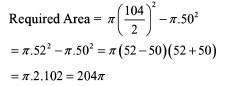Test: Geometry- 2 - Question 10

The dimensions of a rectangular box are in the ratio of 1:2:4 and the difference between the costs of covering it with the cloth and a sheet at the rate of Rs 20 and Rs 20.5 per sq m respectively is Rs 126. Find the dimensions of the box.

Detailed Solution for Test: Geometry- 2 - Question 10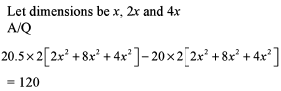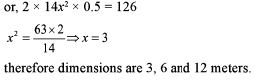Test: Geometry- 2 - Question 11

The ratio of the area of a square inscribed in a semicircle to that of the area of a square inscribed in the circle of the same radius is

Detailed Solution for Test: Geometry- 2 - Question 11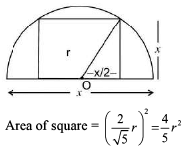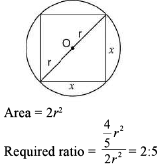Test: Geometry- 2 - Question 12

The ratio of the area of a square to that of the square drawn on the its the diagonal is

Detailed Solution for Test: Geometry- 2 - Question 12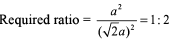Test: Geometry- 2 - Question 13

What is the area of the triangle in which two of its medians 9 cm and 12 cm long intersect at the right angles?

Detailed Solution for Test: Geometry- 2 - Question 13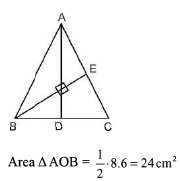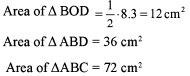Test: Geometry- 2 - Question 14

Four horses are tethered at four comers of a square plot of side 14 m so that the adjacent horses can just reach one another. There is a small circular pond of area 20 m2 at the centre. Find the ungrazed area.

Detailed Solution for Test: Geometry- 2 - Question 14

Total area of plot = 14 * 14 = 196m2
Horses can graze in quarter circle of radius = 7m
Grazed area = 4 * (pie r2)/4 = 154 m2
Area of plot when horses cannot reach = (196 - 154) = 42m2
Ungrazed area = 42 - 20 = 22m2

Test: Geometry- 2 - Question 15

Two sides of a triangle are 4 and 5. Then, for the area of the triangle, which one of the following bounds is the sharpest?

Detailed Solution for Test: Geometry- 2 - Question 15

Let AB = 4 and BC = 5 and AB is perpendicular to BC

then Area = 1/2 AB . AC = 1/2 . 4.5 = 10

## CSAT Preparation for UPSC CSE

72 videos|64 docs|92 tests
 Use Code STAYHOME200 and get INR 200 additional OFF Use Coupon Code
Information about Test: Geometry- 2 Page
In this test you can find the Exam questions for Test: Geometry- 2 solved & explained in the simplest way possible. Besides giving Questions and answers for Test: Geometry- 2, EduRev gives you an ample number of Online tests for practice

## CSAT Preparation for UPSC CSE

72 videos|64 docs|92 tests